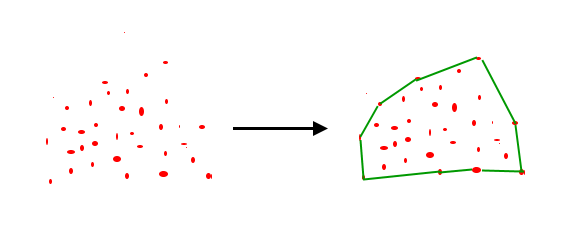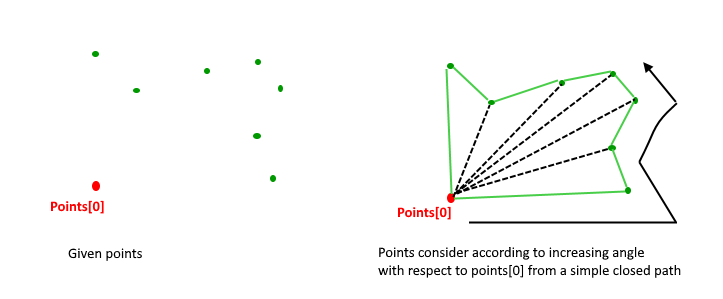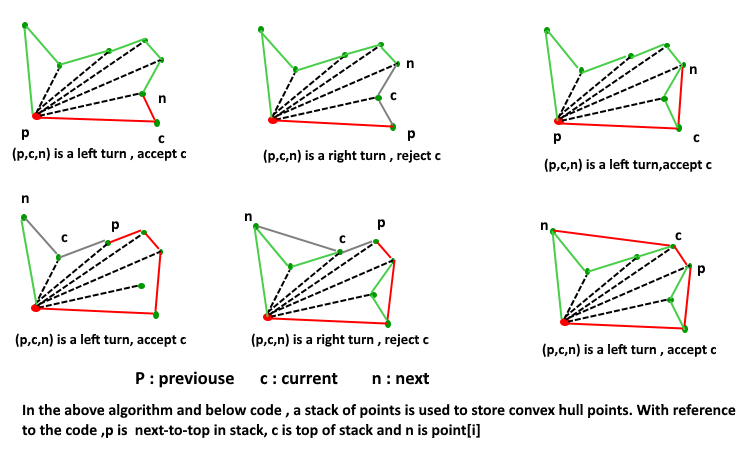Related Articles
Convex Hull | Set 2 (Graham Scan)
• Difficulty Level : Hard
• Last Updated : 08 Mar, 2021

Given a set of points in the plane. the convex hull of the set is the smallest convex polygon that contains all the points of it.We strongly recommend to see the following post first.
How to check if two given line segments intersect?
We have discussed Jarvis’s Algorithm for Convex Hull. The worst case time complexity of Jarvis’s Algorithm is O(n^2). Using Graham’s scan algorithm, we can find Convex Hull in O(nLogn) time. Following is Graham’s algorithm
Let points[0..n-1] be the input array.
1) Find the bottom-most point by comparing y coordinate of all points. If there are two points with the same y value, then the point with smaller x coordinate value is considered. Let the bottom-most point be P0. Put P0 at first position in output hull.
2) Consider the remaining n-1 points and sort them by polar angle in counterclockwise order around points. If the polar angle of two points is the same, then put the nearest point first.
3 After sorting, check if two or more points have the same angle. If two more points have the same angle, then remove all same angle points except the point farthest from P0. Let the size of the new array be m.
4) If m is less than 3, return (Convex Hull not possible)
5) Create an empty stack ‘S’ and push points, points and points to S.
6) Process remaining m-3 points one by one. Do following for every point ‘points[i]’
4.1) Keep removing points from stack while orientation of following 3 points is not counterclockwise (or they don’t make a left turn).
a) Point next to top in stack
b) Point at the top of stack
c) points[i]
4.2) Push points[i] to S
5) Print contents of S
The above algorithm can be divided into two phases.
Phase 1 (Sort points): We first find the bottom-most point. The idea is to pre-process points be sorting them with respect to the bottom-most point. Once the points are sorted, they form a simple closed path (See the following diagram).What should be the sorting criteria? computation of actual angles would be inefficient since trigonometric functions are not simple to evaluate. The idea is to use the orientation to compare angles without actually computing them (See the compare() function below)
Phase 2 (Accept or Reject Points): Once we have the closed path, the next step is to traverse the path and remove concave points on this path. How to decide which point to remove and which to keep? Again, orientation helps here. The first two points in sorted array are always part of Convex Hull. For remaining points, we keep track of recent three points, and find the angle formed by them. Let the three points be prev(p), curr(c) and next(n). If orientation of these points (considering them in same order) is not counterclockwise, we discard c, otherwise we keep it. Following diagram shows step by step process of this phase.Following is C++ implementation of the above algorithm.

## CPP

 `// A C++ program to find convex hull of a set of points. Refer``// https://www.geeksforgeeks.org/orientation-3-ordered-points/``// for explanation of orientation()``#include ``#include ``#include ``using` `namespace` `std;` `struct` `Point``{``    ``int` `x, y;``};` `// A global point needed for  sorting points with reference``// to  the first point Used in compare function of qsort()``Point p0;` `// A utility function to find next to top in a stack``Point nextToTop(stack &S)``{``    ``Point p = S.top();``    ``S.pop();``    ``Point res = S.top();``    ``S.push(p);``    ``return` `res;``}` `// A utility function to swap two points``void` `swap(Point &p1, Point &p2)``{``    ``Point temp = p1;``    ``p1 = p2;``    ``p2 = temp;``}` `// A utility function to return square of distance``// between p1 and p2``int` `distSq(Point p1, Point p2)``{``    ``return` `(p1.x - p2.x)*(p1.x - p2.x) +``          ``(p1.y - p2.y)*(p1.y - p2.y);``}` `// To find orientation of ordered triplet (p, q, r).``// The function returns following values``// 0 --> p, q and r are colinear``// 1 --> Clockwise``// 2 --> Counterclockwise``int` `orientation(Point p, Point q, Point r)``{``    ``int` `val = (q.y - p.y) * (r.x - q.x) -``              ``(q.x - p.x) * (r.y - q.y);` `    ``if` `(val == 0) ``return` `0;  ``// colinear``    ``return` `(val > 0)? 1: 2; ``// clock or counterclock wise``}` `// A function used by library function qsort() to sort an array of``// points with respect to the first point``int` `compare(``const` `void` `*vp1, ``const` `void` `*vp2)``{``   ``Point *p1 = (Point *)vp1;``   ``Point *p2 = (Point *)vp2;` `   ``// Find orientation``   ``int` `o = orientation(p0, *p1, *p2);``   ``if` `(o == 0)``     ``return` `(distSq(p0, *p2) >= distSq(p0, *p1))? -1 : 1;` `   ``return` `(o == 2)? -1: 1;``}` `// Prints convex hull of a set of n points.``void` `convexHull(Point points[], ``int` `n)``{``   ``// Find the bottommost point``   ``int` `ymin = points.y, min = 0;``   ``for` `(``int` `i = 1; i < n; i++)``   ``{``     ``int` `y = points[i].y;` `     ``// Pick the bottom-most or chose the left``     ``// most point in case of tie``     ``if` `((y < ymin) || (ymin == y &&``         ``points[i].x < points[min].x))``        ``ymin = points[i].y, min = i;``   ``}` `   ``// Place the bottom-most point at first position``   ``swap(points, points[min]);` `   ``// Sort n-1 points with respect to the first point.``   ``// A point p1 comes before p2 in sorted output if p2``   ``// has larger polar angle (in counterclockwise``   ``// direction) than p1``   ``p0 = points;``   ``qsort``(&points, n-1, ``sizeof``(Point), compare);` `   ``// If two or more points make same angle with p0,``   ``// Remove all but the one that is farthest from p0``   ``// Remember that, in above sorting, our criteria was``   ``// to keep the farthest point at the end when more than``   ``// one points have same angle.``   ``int` `m = 1; ``// Initialize size of modified array``   ``for` `(``int` `i=1; i S;``   ``S.push(points);``   ``S.push(points);``   ``S.push(points);` `   ``// Process remaining n-3 points``   ``for` `(``int` `i = 3; i < m; i++)``   ``{``      ``// Keep removing top while the angle formed by``      ``// points next-to-top, top, and points[i] makes``      ``// a non-left turn``      ``while` `(S.size()>1 && orientation(nextToTop(S), S.top(), points[i]) != 2)``         ``S.pop();``      ``S.push(points[i]);``   ``}` `   ``// Now stack has the output points, print contents of stack``   ``while` `(!S.empty())``   ``{``       ``Point p = S.top();``       ``cout << ``"("` `<< p.x << ``", "` `<< p.y <<``")"` `<< endl;``       ``S.pop();``   ``}``}` `// Driver program to test above functions``int` `main()``{``    ``Point points[] = {{0, 3}, {1, 1}, {2, 2}, {4, 4},``                      ``{0, 0}, {1, 2}, {3, 1}, {3, 3}};``    ``int` `n = ``sizeof``(points)/``sizeof``(points);``    ``convexHull(points, n);``    ``return` `0;``}`

Output:

```(0, 3)
(4, 4)
(3, 1)
(0, 0) ```

Time Complexity: Let n be the number of input points. The algorithm takes O(nLogn) time if we use a O(nLogn) sorting algorithm.
The first step (finding the bottom-most point) takes O(n) time. The second step (sorting points) takes O(nLogn) time. The third step takes O(n) time. In the third step, every element is pushed and popped at most one time. So the sixth step to process points one by one takes O(n) time, assuming that the stack operations take O(1) time. Overall complexity is O(n) + O(nLogn) + O(n) + O(n) which is O(nLogn).

References:
Introduction to Algorithms 3rd Edition by Clifford Stein, Thomas H. Cormen, Charles E. Leiserson, Ronald L. Rivest
http://www.dcs.gla.ac.uk/~pat/52233/slides/Hull1x1.pdf
Please write comments if you find anything incorrect, or you want to share more information about the topic discussed above.

Attention reader! Don’t stop learning now. Get hold of all the important DSA concepts with the DSA Self Paced Course at a student-friendly price and become industry ready.

My Personal Notes arrow_drop_up